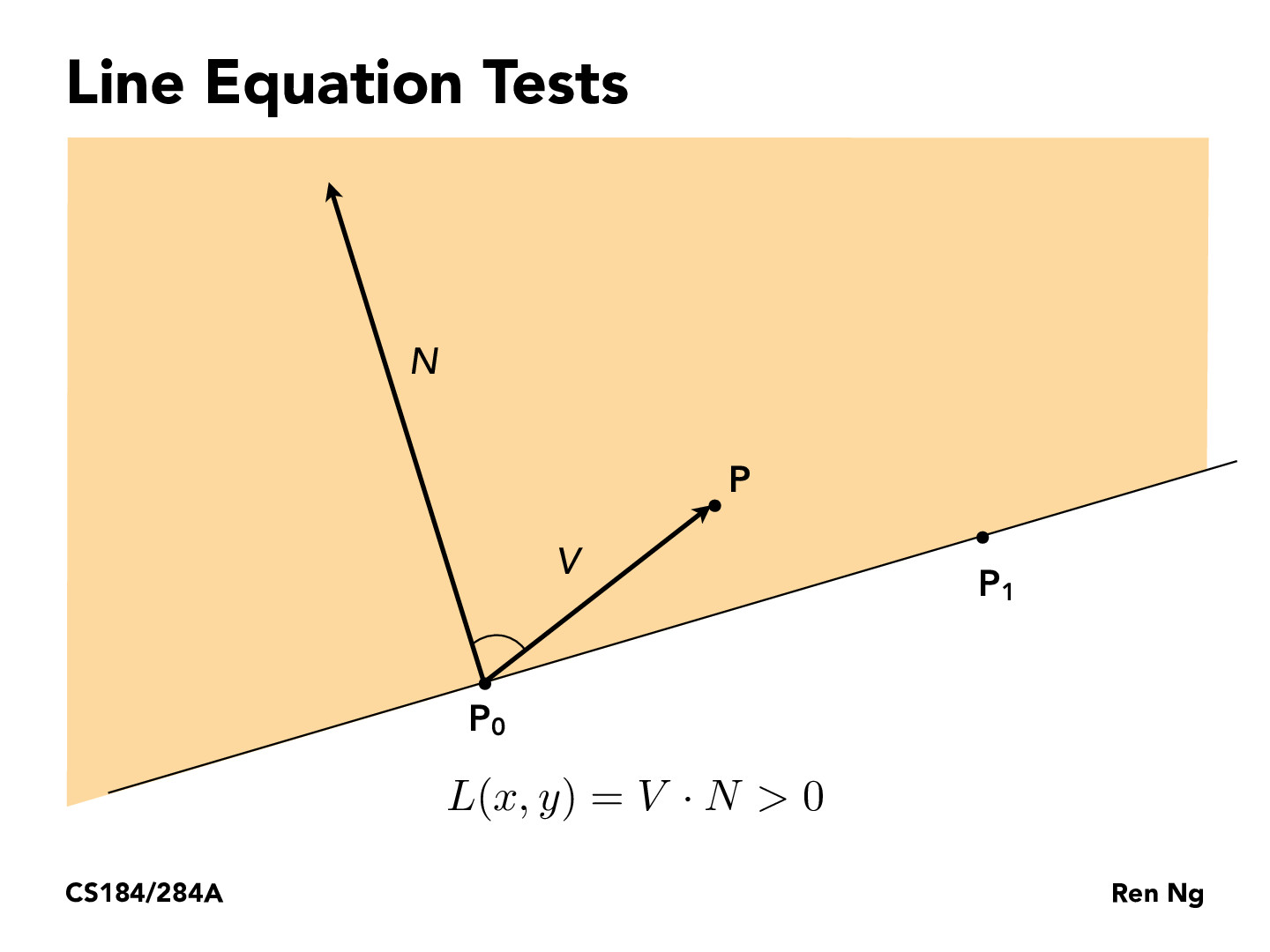Lecture 2: Sampling Triangles (43)shannonhu-144

V dot N is |V||N|cos(theta), where theta is the angle between N and V. If V dot N is greater than 0, then theta must be less than 90, so P must be on the same half-plane as N. If V dot N is 0, then theta is 90, so P must be on the line. If V dot N is negative, then theta is between 90 and 180 (exclusive), so P must be on the opposite half-plane as N.